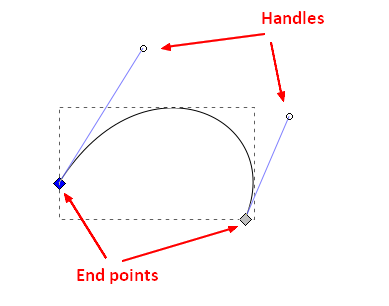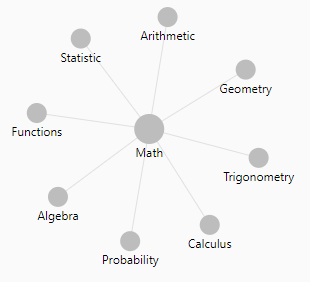# Geometry - Curve (Parabola|Parabolic|Arche)

Parabola's or Parabolic arcs are simply curves or arches.

A parabolic arc is a section of a parabola.

A parabola is the curve graph of a quadratic function.

$y = ax^2 + bx + c$

## Documentation / Reference

Recommended PagesData Visualization - Vector Graphic

A vector graphic is a composed of geometric object and is next to raster (bitmap Image) the second way of representing a image It represents object not by their pixel as in raster (bitmap Image) but by...Geometry - Bezier Curve

Bézier curves are widely used in computer graphics to model smooth curves. TrueType fonts use composite Bézier curves composed of quadratic Bézier curves. Bezier curves are defined by four points:...Image Vector - Path

A path is a graphic object Paths define: an arbitrary shapes, line trajectories, and regions A path may intersect itself and may have disconnected sections and holes. Programs use paths to draw...Mathematics - Quadratic (function|polynomial of degree 2)

In mathematics, a quadratic function, a quadratic polynomial, a polynomial of degree 2, or simply a quadratic, is a polynomial function in one or more variables in which the highest-degree...SVG - Curve (Line)

in SVG. A curved line is just designed with a path element. There are three different path commands that you can use to create smooth curves. bezier curve, an “arc” or part of a circle....